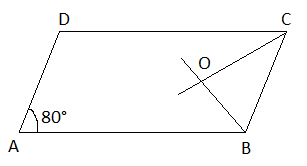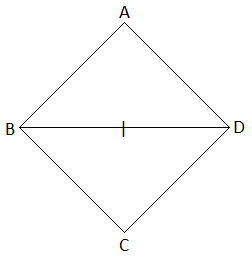# Important Questions for Maths Class 8 Chapter 3 Understanding Quadrilaterals

Important questions for Class 8 chapter 3 – Understanding quadrilaterals are given here. Students who are preparing for final exams can practice these questions to score good marks. All the questions presented here are as per NCERT curriculum or CBSE syllabus.

Understanding quadrilaterals chapter deals with different types of quadrilaterals and their properties. You will also learn to compute the measure of any missing angle of quadrilateral. Let us see some important questions with solutions here.

Students can also reach Important Questions for Class 8 Maths to get important questions for all the chapters here.

## Class 8 Chapter 3 Important Questions

Questions and answers are given here based on important topics of class 8 Maths Chapter 3.

Q.1: A quadrilateral has three acute angles, each measure 80°. What is the measure of the fourth angle?

Solution:

Let x be the measure of the fourth angle of a quadrilateral.

Sum of the four angles of a quadrilateral = 360°

80° + 80° + 80° + x = 360°

x = 360° – (80° + 80° + 80°)

x = 360° – 240°

x = 120°

Hence, the fourth angle is 120°.

Q,2: In a quadrilateral ABCD, the measure of the three angles A, B and C of the quadrilateral is 110°, 70° and 80°, respectively. Find the measure of the fourth angle.

Solution: Let,

A = 110°

B = 70°

C = 80°

D = x

We know that the sum of all internal angles of quadrilateral ABCD is 360°.

A + B+ C+D = 360°

110° + 70° + 80° + x = 360°

260° + x = 360°

x = 360° – 260°

x = 100°

Therefore, the fourth angle is 100°.

Q.3: In a quadrilateral ABCD, ∠D is equal to 150° and ∠A = ∠B = ∠C. Find ∠A, ∠B and ∠C.

Solution: Given,

∠D = 150°

Let ∠A = ∠B = ∠C = x

By angle sum property of quadrilateral,

∠A + ∠B + ∠C + ∠D = 360°

x + x +x+∠D = 360°

3x+∠D = 360°

3x = 360° – ∠D

30 = 360° – 150°

3x = 210°

x = 70°

Hence, ∠A = ∠B = ∠C = 70°.

Q.4: The angles of a quadrilateral are in the ratio of 1 : 2 : 3 : 4. What is the measure of the four angles?

Solution: Given,

The ratio of the angles of quadrilaterals = 1 : 2 : 3 : 4

Let the four angles of the quadrilateral be x, 2x, 3x, and 4x respectively.

The sum of four angles of a quadrilateral is 360°.

Therefore,

x + 2x + 3x + 4x = 360°

10x = 360°

x = 360°/10

x = 36°

Therefore,

First angle = x = 36°

Second angle = 2x = 2 × 36 = 72°

Third angle = 3x = 3 × 36 = 108°

Fourth angle = 4x = 4 × 36 = 144°

Hence, the measure of four angles is 36°, 72°, 108° and 144°.

(i) which of them have their diagonals bisecting each other?

(ii) which of them have their diagonal perpendicular to each other?

(iii) which of them have equal diagonals?

Solution:

(i) Diagonals bisect each other in:

• Parallelogram
• Rhombus
• Rectangle
• Square
• Kite

(ii) Diagonals are perpendicular in:

• Rhombus
• Square
• Kite

(iii) Diagonals are equal to each other in:

• Rectangle
• Square

Q. 6: Adjacent sides of a rectangle are in the ratio 5 : 12, if the perimeter of the rectangle is 34 cm, find the length of the diagonal.

Solution:
Given,
Ratio of the adjacent sides of the rectangle = 5 : 12
Let 5x and 12x be the two adjacent sides.
We know that the sum of all sides of a rectangle is equal to its perimeter.
Thus,
5x + 12x + 5x + 12x = 34 cm (given)
34x = 34
x = 34/34
x = 1 cm
Therefore, the adjacent sides are 5 cm and 12 cm respectively.
i.e. l = 12 cm, b = 5 cm
Length of the diagonal = √(l2 + b2)
= √(122 + 52)
= √(144 + 25)
= √169
= 13 cm
Hence, the length of the diagonal is 13 cm.

Q. 7: The opposite angles of a parallelogram are (3x + 5)° and (61 – x)°. Find the measure of four angles.

Solution:
Given,
(3x + 5)° and (61 – x)° are the opposite angles of a parallelogram.
We know that the opposite angles of a parallelogram are equal.
Therefore,
(3x + 5)° = (61 – x)°
3x + x = 61° – 5°
4x = 56°
x = 56°/4
x = 14°
⇒ 3x + 5 = 3(14) + 5 = 42 + 5 = 47
61 – x = 61 – 14 = 47
The measure of angles adjacent to the given angles = 180° – 47° = 133°
Hence, the measure of four angles of the parallelogram are 47°, 133°, 47°, and 133°.

Q. 8: ABCD is a parallelogram with ∠A = 80°. The internal bisectors of ∠B and ∠C meet each other at O. Find the measure of the three angles of ΔBCO.

Solution:
Given,
∠A = 80°We know that the opposite angles of a parallelogram are equal.
∠A = ∠C = 80°
And
∠OCB = (1/2) × ∠C
= (1/2) × 80°
= 40°
∠B = 180° – ∠A (the sum of interior angles on the same side of the transversal is 180)
= 180° – 80°
= 100°
Also,
∠CBO = (1/2) × ∠B
= (1/2) × 100°
= 50°
By the angle sum property of triangle BCO,
∠BOC + ∠OBC + ∠CBO = 180°
∠BOC = 180° – (∠OBC + CBO)
= 180° – (40° + 50°)
= 180° – 90°
= 90°
Hence, the measure of all the three angles of a triangle BCO is 40°, 50° and 90°.

Q. 9: Find the measure of all four angles of a parallelogram whose consecutive angles are in the ratio 1 : 3.

Solution:
Given,
The ratio of two consecutive angles of a parallelogram = 1 : 3
Let x and 3x be the two consecutive angles.
We know that the sum of interior angles on the same side of the transversal is 180°.
Therefore, x + 3x = 180°
4x = 180°
x = 180°/4
x = 45°
⇒ 3x = 3(45°) = 135°
Thus, the measure of two consecutive angles is 45° and 135°.
As we know, the opposite angles of a parallelogram are equal.
Hence, the measure of all the four angles is 45°, 135°, 45°, and 135°.

Q. 10: A diagonal and a side of a rhombus are of equal length. Find the measure of the angles of the rhombus.

Solution:
Let ABCD be the rhombus.
Thus, AB = BC = CD = DAGiven that a side and diagonal are equal.
AB = BD (say)
Therefore, AB = BC = CD = DA = BD
Now, all the sides of a triangle ABD are equal.
Therefore, ΔABD is an equilateral triangle.
Similarly,
ΔBCD is also an equilateral triangle.
Thus, ∠A = ∠ABD = ∠ADB = ∠DBC = ∠C = ∠CDB = 60°
∠B = ∠ABD + ∠DBC = 60° + 60° = 120°
And
∠D = ∠ADB + ∠CDB = 60° + 60° = 120°
Hence, the angles of the rhombus are 60°, 120°, 60° and 120°.

### Extra Practice Questions For Class 8 Maths Chapter 3 Understanding Quadrilateral

1. How many sides do a regular polygon have, if the measure of an exterior angle is given as 24° ?

2. What is the measure of each exterior angle of a regular polygon of 15 sides?
(a) 30°
(b) 45°
(c) 60°
(d) 24°

3.  When the sum of the internal angles of a polygon is 10 right angles, then how many sides does it have?
(a) 6
(b) 5
(c) 8
(d) 7

4. Select a false statement from those given below:
(a) A square is a rectangle that has equal adjacent sides
(b) A square is a rhombus whose one angle is a right angle
(c) The diagonals of a square bisect each other at right angles
(d) The diagonals of a square do not divide the whole square into four equal parts.

5. When one angle of a parallelogram is a right angle, then what is the name of the quadrilateral?
(a) kite
(b) rectangle
(c) rhombus
(d) square

1. Shabbir Mustafa

Wow helped ma a lot. Thank you!!!!

2. SWARUJ

very nice andd easy for me but enjoyed it thanks

3. Sujir Salian

4. Devanshu

Good questions

5. Thank you so much

6. It helped me toooooooooooooooo much thank you BYJU’S

7. omg thanks soo much!
and im a byjus subscriber!

8. akshaya

Thanks byjus.
.It helped me a lot.

9. Nice questions

10. Roshani Panchariya

IT WAS EXXTREMLY EASY THANKS BYJUS BY SOLVING THIS I HAVE A BIT OF CONFIDENCE THANKS A LOT AND THE FACILITY OF START THE CHAT IN BOTTOM RIGHT CORNER IS EXTREMLY HELPFUL IN WICH OUR DOUBTS ARE SOLVED IN FREE OF COST